# SSAT Elementary Level Math : How to find the whole from the part

## Example Questions

### Example Question #31 : Describing And Comparing Measurable Attributes

Which shape is smaller?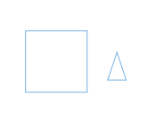The square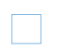The triangle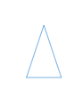They are the same size

The triangleExplanation:

The triangle is smaller than the square.

### Example Question #82 : How To Find The Whole From The Part

Which shape is smaller?The circle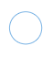They are the same size

The squareThe circleExplanation:

The circle is smaller than the square.

### Example Question #83 : How To Find The Whole From The Part

Which shape is smaller?The triangle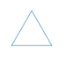The circleThey are both the same size

The circleExplanation:

The circle is smaller than the triangle.

### Example Question #84 : How To Find The Whole From The Part

Which shape is smaller?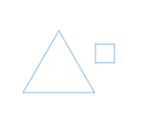The squareThey are the same size

The triangleThe squareExplanation:

The square is smaller than the triangle.

### Example Question #85 : How To Find The Whole From The Part

Which shape is smaller?They are the same size

The triangleThe rectangleThe triangleExplanation:

The triangle is smaller than the rectangle.

### Example Question #86 : How To Find The Whole From The Part

Which line is shorter?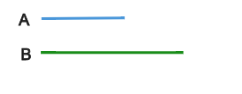They are the same length

Line B

Line A

Line A

Explanation:

Line A is shorter than line B.

### Example Question #41 : Describing And Comparing Measurable Attributes

Which line is shorter?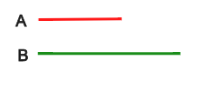They are the same length

Line B

Line A

Line A

Explanation:

Line A is shorter than line B.

### Example Question #42 : Describing And Comparing Measurable Attributes

Which line is shorter?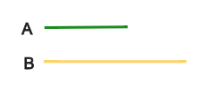Line A

They are the same length

Line B

Line A

Explanation:

Line A is shorter than line B.

### Example Question #89 : How To Find The Whole From The Part

Which line is shorter?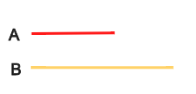Line B

Line A

They are the same length

Line A

Explanation:

Line A is shorter than line B.

### Example Question #90 : How To Find The Whole From The Part

Which line is shorter?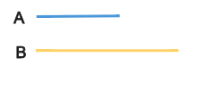Line B

They are the same length

Line A